maths > trigonometry

Trigonometric Ratios for Standard Angles

what you'll learn...

Overview

»  Quickly follow the angle description to calculate the ratios. No need to memorize.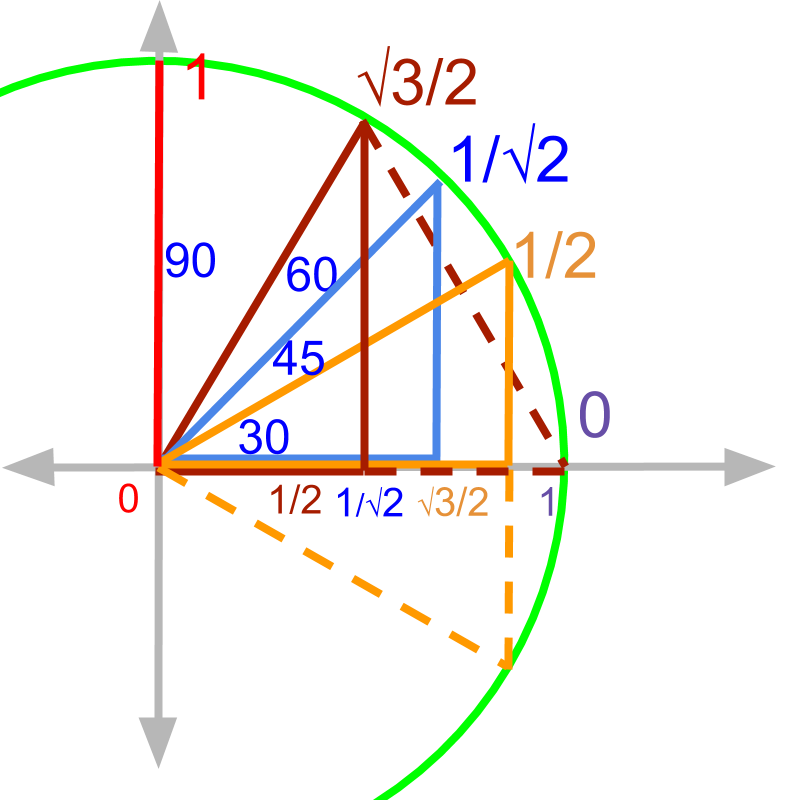isosceles right triangle : ${45}^{\circ }$${45}^{\circ}$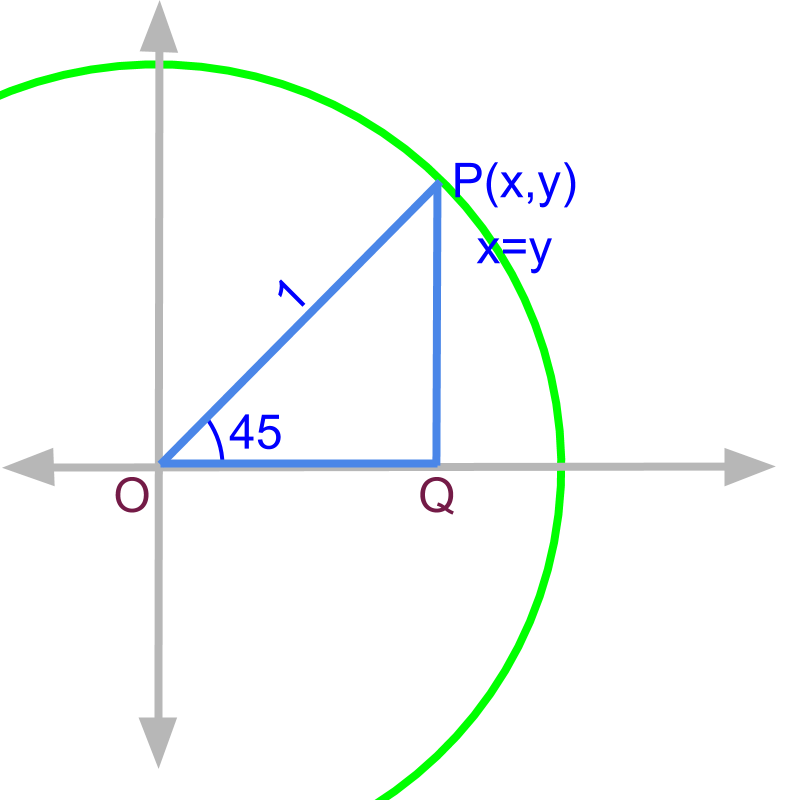Given the right angled triangle $\Delta OPQ$$\Delta O P Q$, and $\angle POQ={45}^{\circ }$$\angle P O Q = {45}^{\circ}$.

$\overline{OP}=1$$\overline{O P} = 1$
$\overline{OQ}=\overline{PQ}=x=y$$\overline{O Q} = \overline{P Q} = x = y$
${\overline{OQ}}^{2}+{\overline{PQ}}^{2}={\overline{OP}}^{2}=1$${\overline{O Q}}^{2} + {\overline{P Q}}^{2} = {\overline{O P}}^{2} = 1$

$2{x}^{2}=1$$2 {x}^{2} = 1$

The point P is $\left(\frac{1}{\sqrt{2}},\frac{1}{\sqrt{2}}\right)$$\left(\frac{1}{\sqrt{2}} , \frac{1}{\sqrt{2}}\right)$.

It is proven that point $P\left(\frac{1}{\sqrt{2}},\frac{1}{\sqrt{2}}\right)$$P \left(\frac{1}{\sqrt{2}} , \frac{1}{\sqrt{2}}\right)$. That is,

opposite side $\overline{PQ}=\frac{1}{\sqrt{2}}$$\overline{P Q} = \frac{1}{\sqrt{2}}$

adjacent side $\overline{OQ}=\frac{1}{\sqrt{2}}$$\overline{O Q} = \frac{1}{\sqrt{2}}$

hypotenuse $\overline{OP}=1$$\overline{O P} = 1$

${\mathrm{sin}45}^{\circ }=\frac{1}{\sqrt{2}}$$\sin {45}^{\circ} = \frac{1}{\sqrt{2}}$

${\mathrm{cos}45}^{\circ }=\frac{1}{\sqrt{2}}$$\cos {45}^{\circ} = \frac{1}{\sqrt{2}}$

${\mathrm{tan}45}^{\circ }=1$$\tan {45}^{\circ} = 1$

Do not memorize the trigonometric ratios, it is easy to work out the lengths of the three sides of a triangle of standard angles.

Once we know the lengths of the three sides, it is easy to work out the trigonometric ratios.

For example, ${\mathrm{cot}45}^{\circ }$$\cot {45}^{\circ}$ is $1$$1$ (by finding ratio adjacent by opposite side).

equilateral triangle : ${60}^{\circ }$${60}^{\circ}$ and ${30}^{\circ }$${30}^{\circ}$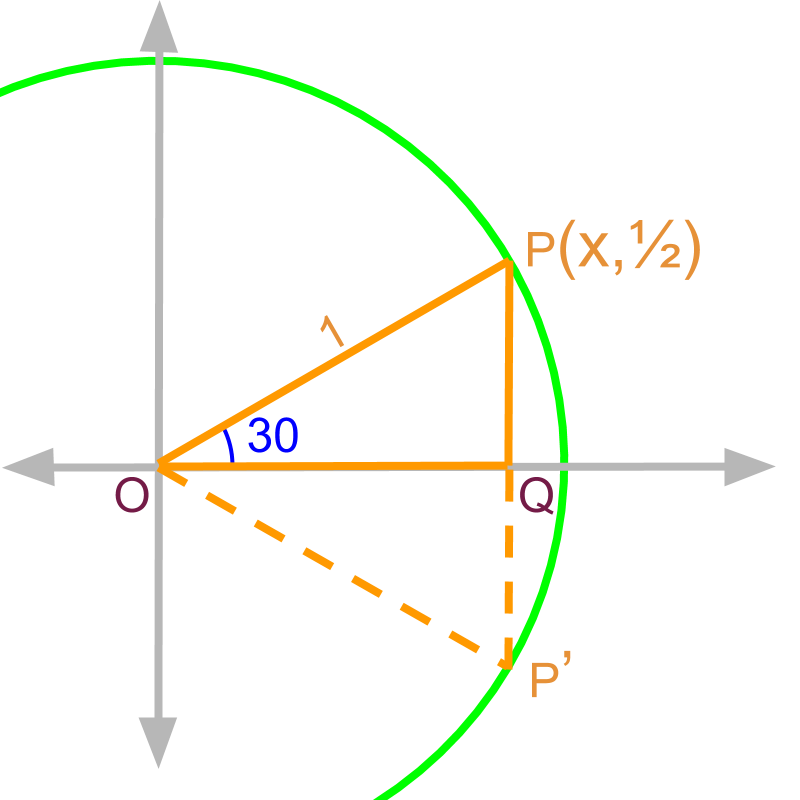Given the right angled triangle $\Delta OPQ$$\Delta O P Q$, and $\angle POQ={30}^{\circ }$$\angle P O Q = {30}^{\circ}$.

$\overline{OP}=1$$\overline{O P} = 1$
Construct an equilateral triangle $\Delta OP\prime P$.
From this, it is derived that $\overline{PQ}=\frac{1}{2}$$\overline{P Q} = \frac{1}{2}$.
${\overline{OQ}}^{2}+{\overline{PQ}}^{2}={\overline{OP}}^{2}=1$${\overline{O Q}}^{2} + {\overline{P Q}}^{2} = {\overline{O P}}^{2} = 1$

${x}^{2}+{\left(\frac{1}{2}\right)}^{2}=1$${x}^{2} + {\left(\frac{1}{2}\right)}^{2} = 1$

The point $P$$P$ is $\left(\frac{\sqrt{3}}{2},\frac{1}{2}\right)$$\left(\frac{\sqrt{3}}{2} , \frac{1}{2}\right)$.

It is proven that point $P\left(\frac{\sqrt{3}}{2},\frac{1}{2}\right)$$P \left(\frac{\sqrt{3}}{2} , \frac{1}{2}\right)$. That is,

opposite side $\overline{PQ}=\frac{1}{2}$$\overline{P Q} = \frac{1}{2}$

adjacent side $\overline{OQ}=\frac{\sqrt{3}}{2}$$\overline{O Q} = \frac{\sqrt{3}}{2}$

hypotenuse $\overline{OP}=1$$\overline{O P} = 1$

${\mathrm{sin}30}^{\circ }=\frac{1}{2}$$\sin {30}^{\circ} = \frac{1}{2}$

${\mathrm{cos}30}^{\circ }=\frac{\sqrt{3}}{2}$$\cos {30}^{\circ} = \frac{\sqrt{3}}{2}$

${\mathrm{tan}30}^{\circ }=\frac{1}{\sqrt{3}}$$\tan {30}^{\circ} = \frac{1}{\sqrt{3}}$

It is noted that one does not need to memorize the trigonometric ratios, it is easy to work out the lengths of the three sides of a triangle of standard angles. Once we know the lengths of the three sides, it is easy to work out the trigonometric ratios.

For example, ${\mathrm{sec}30}^{\circ }=\frac{2}{\sqrt{3}}$$\sec {30}^{\circ} = \frac{2}{\sqrt{3}}$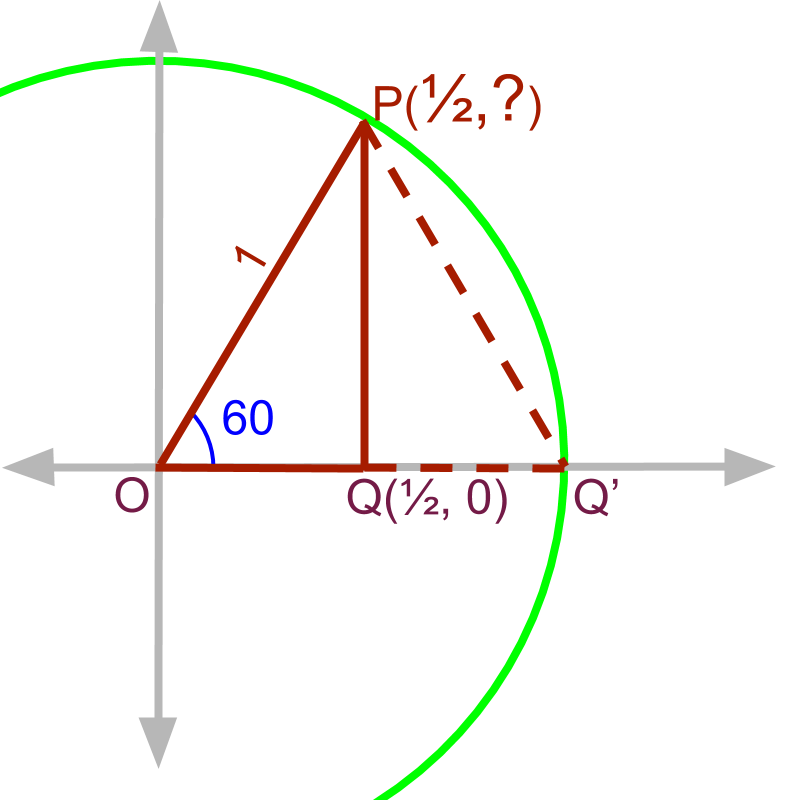Given the right angled triangle $\Delta OPQ$$\Delta O P Q$, and $\angle POQ={60}^{\circ }$$\angle P O Q = {60}^{\circ}$.

$\overline{OP}=1$$\overline{O P} = 1$
Construct an equilateral triangle $\Delta OQ\prime P$.
From this, it is derived that $\overline{OQ}=\frac{1}{2}$$\overline{O Q} = \frac{1}{2}$.
${\overline{OQ}}^{2}+{\overline{PQ}}^{2}={\overline{OP}}^{2}=1$${\overline{O Q}}^{2} + {\overline{P Q}}^{2} = {\overline{O P}}^{2} = 1$

${\left(\frac{1}{2}\right)}^{2}+{y}^{2}=1$${\left(\frac{1}{2}\right)}^{2} + {y}^{2} = 1$

The point $P$$P$ is $\left(\frac{1}{2},\frac{\sqrt{3}}{2}\right)$$\left(\frac{1}{2} , \frac{\sqrt{3}}{2}\right)$.

It is proven that point $P\left(\frac{1}{2},\frac{\sqrt{3}}{2}\right)$$P \left(\frac{1}{2} , \frac{\sqrt{3}}{2}\right)$. That is,

opposite side $\overline{PQ}=\frac{\sqrt{3}}{2}$$\overline{P Q} = \frac{\sqrt{3}}{2}$

adjacent side $\overline{OQ}=\frac{1}{2}$$\overline{O Q} = \frac{1}{2}$

hypotenuse $\overline{OP}=1$$\overline{O P} = 1$

${\mathrm{sin}60}^{\circ }=\frac{\sqrt{3}}{2}$$\sin {60}^{\circ} = \frac{\sqrt{3}}{2}$

${\mathrm{cos}60}^{\circ }=\frac{1}{2}$$\cos {60}^{\circ} = \frac{1}{2}$

${\mathrm{tan}60}^{\circ }=\sqrt{3}$$\tan {60}^{\circ} = \sqrt{3}$

It is repeated that one does not need to memorize the trigonometric ratios, it is easy to work out the lengths of the three sides of a triangle of standard angles and thus the ratios.

For example, the $\text{cosec}{60}^{\circ }=\frac{2}{\sqrt{3}}$$\textrm{\cos e c} {60}^{\circ} = \frac{2}{\sqrt{3}}$

Right Triangle with ${0}^{\circ }$${0}^{\circ}$ and ${90}^{\circ }$${90}^{\circ}$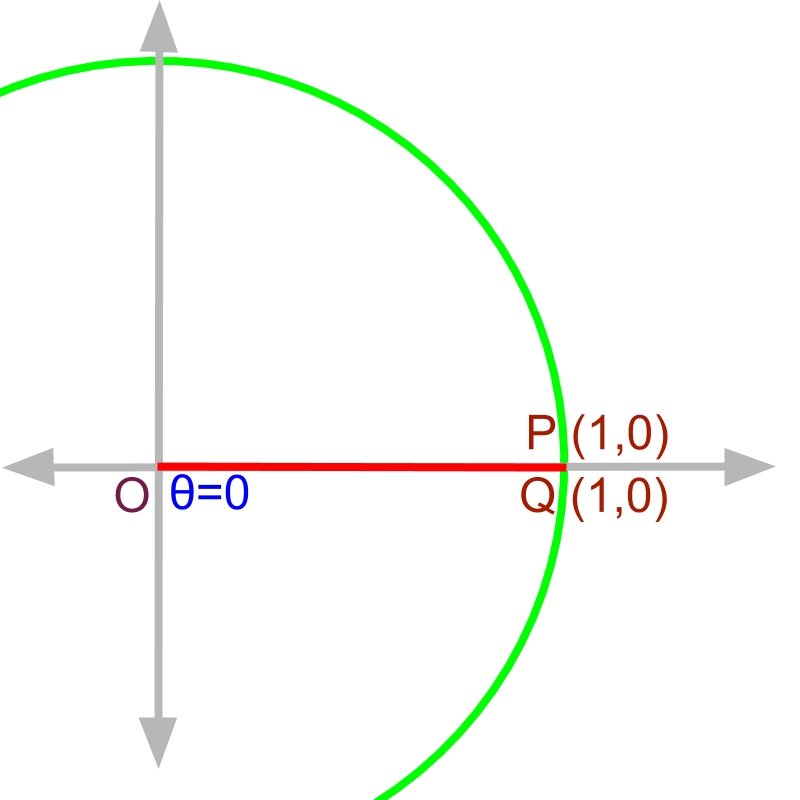Given the right angled triangle $\Delta OPQ$$\Delta O P Q$, and $\angle POQ={0}^{\circ }$$\angle P O Q = {0}^{\circ}$.

$\overline{OQ}=\overline{OP}=1$$\overline{O Q} = \overline{O P} = 1$

The point $P$$P$ is $\left(1,0\right)$$\left(1 , 0\right)$

It is proven that point $P\left(1,0\right)$$P \left(1 , 0\right)$. That is,

opposite side $\overline{PQ}=0$$\overline{P Q} = 0$

adjacent side $\overline{OQ}=1$$\overline{O Q} = 1$

hypotenuse $\overline{OP}=1$$\overline{O P} = 1$

${\mathrm{sin}0}^{\circ }=0$$\sin {0}^{\circ} = 0$

${\mathrm{cos}0}^{\circ }=1$$\cos {0}^{\circ} = 1$

${\mathrm{tan}0}^{\circ }=0$$\tan {0}^{\circ} = 0$

It might not be intuitive, but it is possible to work out the lengths of the three sides of a triangle of ${0}^{\circ }$${0}^{\circ}$ degree and work out the trigonometric ratios without memorizing them.

For example, ${\mathrm{sec}0}^{\circ }=1$$\sec {0}^{\circ} = 1$.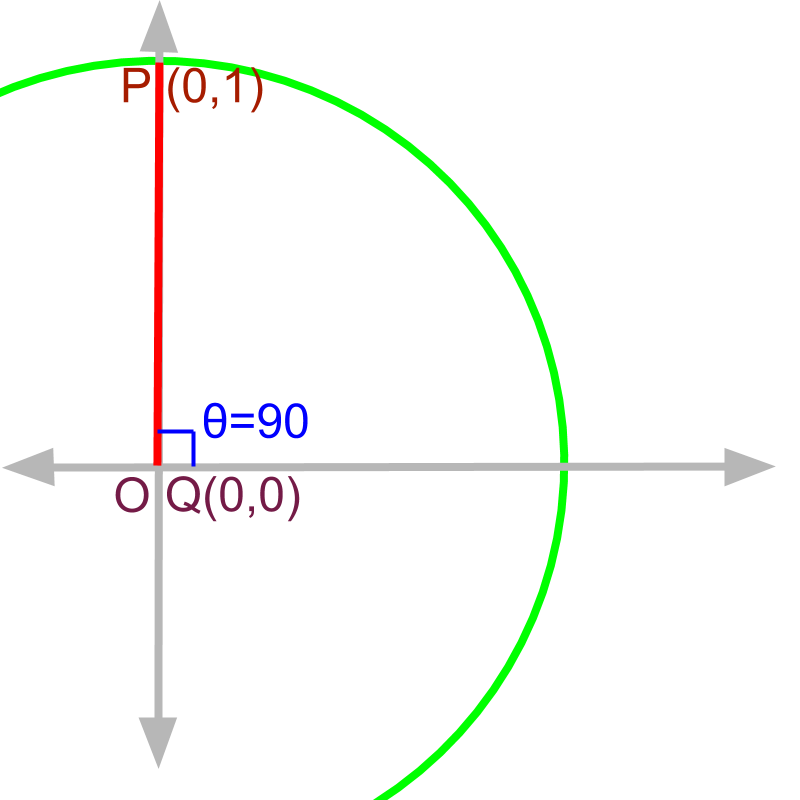Given the right angled triangle $\Delta OPQ$$\Delta O P Q$, and $\angle POQ={90}^{\circ }$$\angle P O Q = {90}^{\circ}$.

$\overline{OP}=\overline{QP}=1$$\overline{O P} = \overline{Q P} = 1$

The point $P$$P$ is $\left(0,1\right)$$\left(0 , 1\right)$.

It is proven that point $P\left(0,1\right)$$P \left(0 , 1\right)$. That is,

opposite side $\overline{PQ}=1$$\overline{P Q} = 1$

adjacent side $\overline{OQ}=0$$\overline{O Q} = 0$

hypotenuse $\overline{OP}=1$$\overline{O P} = 1$

${\mathrm{sin}90}^{\circ }=1$$\sin {90}^{\circ} = 1$

${\mathrm{cos}90}^{\circ }=0$$\cos {90}^{\circ} = 0$

${\mathrm{tan}90}^{\circ }=\frac{1}{0}$$\tan {90}^{\circ} = \frac{1}{0}$

Another triangle that is not very intuitive, but it is possible to work out the lengths of the three sides of a triangle of ${90}^{\circ }$${90}^{\circ}$ and work out the trigonometric ratios without memorizing them.

For example, $\text{cosec}{90}^{\circ }=1$$\textrm{\cos e c} {90}^{\circ} = 1$.

SummaryTrigonometric ratios for all standard angles is captured in the figure.

When a trigonometric ratio is required for an angle, quickly work out the $x,y$$x , y$ for the angle. And sine is chord, cosine is the chord on the complementary angle, tan is the tangent etc. With a little bit of practice, this becomes fast and easy. I hope you do not memorize a table which you will eventually forget. If you can work out from the first principles, the information will last a lot longer. With a little bit of practice, students can recall the ratios as fast as when memorized.

Trigonometric Ratios of Standard Angles:

When a trigonometric ratio is required for an angle, quickly work out the $x,y$$x , y$ for the angle.

Outline

The outline of material to learn "Basics of Trigonometry" is as follows.

•   Detailed outline of "trigonometry".

→   Basics - Angles

→   Basics - Triangles

→   Importance of Right Angled Triangle

→   Trigonometric Ratio (Basics)

→   Triangular Form of Trigonometric Ratios

→   Introduction to Standard Angles

→   Trigonometric Ratio of Standard Angles

→   Trigonometric Identities

→   Trigonometric Ratios of Complementary Angles